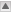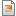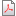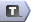Laboratoire Interdisciplinaire de Physique (LIPhy), Grenoble, FRANCE

## Research interests

SUPERCOOLED LIQUIDS AND THE GLASS TRANSITION

In this field my research activity has been devoted to the study of amorphous order in supercooled liquids, mainly through numerical simulations (papers [2-6] below) but also with analytical methods (paper [0-1]), e.g. the study of istantonic solutions of a replica field theory. I am a proficient programmer in C, the programming language that I used for writing codes for the simulations of both lattice (spin models) and off-lattice (liquids) models of glass-formers. My main contributions to the field are the following. First I want to mention the numerical measure, presented in , of the distribution of the overlap order parameter, which is associated to the glass-transition, in the deeply supercooled regime of a glass-forming liquid. The deeply supercooled regime is when a viscous liquid is cooled far below its melting temperature without cristallizing: a regime which is particularly delicate to handle in numerical simulations. Our numerical results, obtained for a realistic 3D glass-former, suggest the existence of a transition at low temperatures from a low-overlap (liquid) phase to a high-overlap (glassy) phase, in agreement with standard mean-field predictions. The overlap'' is the order parameter which in the field-theoretical formulation characterizes the glass transition. Another relevant result that I have obtained in this field was due to a collaboration with G. Biroli: by finding non-perturbative solutions of the appropriate replica field-theory we clarified which is the role of geometric confinement in systems which usually become glassy by lowering the temperature. We obtained the results of  working on a toy model where exact calculations are possible: this notwidthstanding, we expect them to be particularly relevant for a better understanding of the physics of glass-forming materials confined in porous matrices. Working together with people expert in the field of the glass transition I had indeed the chance to learn several different methods, not only numerical. In the paper , which is still in preparation, we approached the problem of the glass transition in a lattice spin model by means of several techniques. These techniques include the use of algorithms developed to determine the solvability of constrained satisfaction problems, an issue that is well know to be related to the problem of the glass transition; also, we made use of the cavity method and the Belief Propagation equations, which allows one to exactly calculate all thermodynamic potentials for systems on random graph geometries.

 The Random-Diluted Triangular Plaquette Model: study of phase transitions in a Kinetically Constrained Model
S. Franz, G. Gradenigo, S. Spigler, in preparation .

 Confinement as a tool to probe amorphous order
C. Cammarota, G. Gradenigo, G. Biroli Phys. Rev. Lett. ( Editor's suggestion )111, 107801 (2013).

 Static correlations functions and domain walls in glass-forming liquids: the case of a sandwich geometry
G. Gradenigo, R. Trozzo, A. Cavagna, T. Grigera, P. Verrocchio J. Chem. Phys. 138, 12A509 (2013)

 The ratchet effect in an ageing glass
Gradenigo G., Sarracino A., Villamaina D., Grigera T. S. and Puglisi A. J.Stat.Mech. (2010) L12002.
(http://iopscience.iop.org/1742-5468/2010/12/L12002?fromSearchPage=true)

 Phase-Separation Perspective on Dynamic Heterogeneities in Glass-Forming Liquids
Cammarota C., Cavagna A., Giardina I., Gradenigo G., Grigera T.S., Parisi G. and Verrocchio P. PRL, 105, 055703 (2010).
(http://prl.aps.org/abstract/PRL/v105/i5/e055703)

 Evidence for a spinodal limit of amorphous excitations in glassy systems
Cammarota C., Cavagna A., Gradenigo G., Grigera T.S. and Verrocchio P. J.Stat.Mech. (2009) L12002.
(http://iopscience.iop.org/1742-5468/2009/12/L12002?fromSearchPage=true)

 Numerical determination of the exponents controlling the relationship between time, length, and temperature in glass-forming liquids
Cammarota C., Cavagna A., Gradenigo G., Grigera T.S. and Verrocchio P. J.Chem.Phys. 131, 194901 (2009).
(http://jcp.aip.org/resource/1/jcpsa6/v131/i19/p194901_s1)

ANOMALOUS DIFFUSION

The properties of the erratic motion of a tracer particle, like a grain of dust within a glass of water, are well known when the environment'' is a homogeneous and equilibrium one: in this case one finds the well-known Brownian motion characterized by a mean squared displacement of the intruder increasing linearly with the observational time, < X^2(t)> ~ t. In this situation it is also well know that when the erratic motion of the tracer particle is subjected to a small external field $\epsilon$ there is a finite drift in the direction of the field: <X(t)> ~\epsilon t.

What is more intriguing, and has been the object of several of my publications [7,8,9,10], is the effect of an external perturbation in the presence of anomalous diffusion: anomalous diffusion is when the mean squared displacement of our tracer particles in the host medium grows like <X^2(t)> ~t^{\nu}, with $\nu\neq 1$. Most often these are situations where the standard tools of equilibrium statistical mechanics cannot be used and one must resort on more general approaches, e.g. the study of the Master Equation governing the process, which are well defined even in absence of an equilibrium environment.

The more interesting result that I have obtained on this subject is discussed in  and  and concerns the relation between the shape of probability distributions of displacements in presence of an external field and the exponent $\nu$ which characterize the anomaly in the diffusional properties. The models we studied are the Continuous Time Random Walk model with trapping, which shows subdiffusion ($\nu<1$), and the Levy Walk model, which shows superdiffusion ($\nu>1$). The study of the interplay between the action of an external field and anomalous diffusive properties is quite of interest because anomalous diffusion is present in a large class of systems, ranging from biological ones to inhomogeous materials.

 Scaling properties of field-induced superdiffusion in Continous Time Random Walks
R. Burioni, G. Gradenigo, A. Sarracino, A. Vezzani, A. Vulpiani, Theor. Phys. 62, 514 (2014).

 Rare events and scaling properties in field-induced anomalous dynamics
R. Burioni, G. Gradenigo, A. Sarracino, A. Vezzani, A. Vulpiani, J. Stat. Mech. P09022 (2013).

 Einstein relation in superdiffusive systems
Gradenigo G., Sarracino A., Villamaina D. and Vulpiani A.
J.Stat.Mech. (2012) L06001
(http://arxiv.org/abs/1205.6621=true)

 On anomalous diffusion and the out of equilibrium response function in one-dimensional models
Villamaina D., Sarracino A., Gradenigo G., Puglisi A. and Vulpiani A.
J.Stat.Mech. (2011) L01002.
(http://iopscience.iop.org/1742-5468/2011/01/L01002?fromSearchPage=true)

FLUCTUATION THEOREM

The most universal property that has been discovered in driven stationary system is the Fluctuation Relation: this is a symmetry of the probability distribution of the entropy produced per unit time, $\sigma$, which reads as $\log[P(\sigma)/P(-\sigma)]=\sigma$. In papers  and  of the list below I realized, in collaboration with people in Rome, a detailed study of a toy model of a gas such that exact results are available on $P(\sigma)$ and therefore the interplay between the Fluctuation Relation and other phyisical properties of the system can be discussed with remarkable precision. The model of  and  is a coarse-grained toy model of a gas where deterministic and stochastic ingredients are coexisting. The model is characterized by inelastic collisions among particles alternated by a deterministic motion which, in presence of an external field, is ballistic: this is the stochastic Lorentz gas. Due to the inelastic collisions and the external field the system is out-of-equilibrium'': nevertheless, due to the simplicity of the ingredients concourring in the description, the related Boltzmann equation can be solved and the properties of the system determined with accuracy. The Boltzmann equation is a very general one for the probability of the microstates: it requires the only assuption of molecular chaos'' and holds also for out-of-equilibrium systems. The unknown of the Boltzmann equation is indeed the probability distribution of microstates: the equation is nonlinear and in general is not possible to solve it exactly. Nevertheless, there are systems simple enough that the equation becomes linear and can also be solved, like the one we studied in ,. One of the properties which are more often studied in systems like the stochastic Lorentz gas is the probability of very unlikely events. While in an equilibrium system the average entropy produced per unit time is zero, within an out-of-equilibrium system this entropy is positive. Indeed one can assume as an equivalent definition of being out-of-equilibrium'' that of having a positive entropy production. We must then say that a positive entropy production is an \emph{average} property of \emph{macroscopic} systems. When a system is small, there can be rare fluctuations leading to a \emph{negative} entropy production. There is a branch of the theory of probability that is called Large Deviation theory and which is dealth with the study of these rare events. In  and  we used the stochastic Lorentz gas as a benchmark to compare the predictions of the Large Deviation theory on $P(\sigma)$ with the symmetry properties of $P(\sigma)$ which correspond to the Fluctuation Relation.

 Fluctuation relations without uniform large deviations
G. Gradenigo, A. Sarracino, A. Puglisi, H. Touchette, J. Phys. A: Math. Theor. 46, 335002, (2013).

 Non-equilibrium fluctuations in a driven stochastic Lorenz gas
G. Gradenigo, U. Marini Bettolo Marconi, A. Puglisi, and A. Sarracino, Phys. Rev. E 85, 031112 (2012).

GRANULAR FLUIDS

Granular fluids are a paradigmatic example of systems which cannot be described with the standard tools of stastistical mechanics, namely with the Boltzmann distribution of microstates. A fluid, which can be more or less dense, is said granular'' when its elementary components, which are usually mesoscopic beads, have inelastic collisions: this means that some energy is lost within every collision. Clearly, in order to prevent all the energy present in the system to be sucked away by the collisions, leaving the system in a quiet death, the characterization of a granular gas is usually provided also specifying the mechanism by which energy is supplied to the systems. Such a mechanism could be a homogeneous driving of all the particles, like the one discussed in several of my papers on that subject [14,17-19], but may also act just across the boundaries of the system. The continuous flux of energy across the system allows the granular gas to approach a non-equilibrium stationary state (NESS) where the distribution of microstates becomes time-independent and is of course different from the Boltzmann one. Granular fluids display several interesting phenomena that are totally absent in equilibrium fluids and that I studied during my three year post-doc in the TNT group.

Among these it is worthwile to mention the behaviour of correlations, i.e. of cooperativity, between the elementary degree of the system, which differ remarkably with respect to equilibrium fluids. In equilibrium systems it happens that scale-free correlations, namely the simultaneous cooperation of all the degrees of freedom of the system, can be found only in the vicinity of a phase transition. On the contrary in granular gases and in other out-of-equilibrium systems this high degree of cooperativity can be found even far from critical points, and is just due to the out-of-equilibrium'' nature of the system.

The intriguing point abount correlations in granular fluids to which I dedicated most of my work on granular system is the precise characterization of how the range of correlations depend on the energy injection mechanism exploited to keep the system in a stationary state. This dependence of correlations on the energy injection mechanism is the main point that I underlined in the study of the correlations among the velocities of granular beads presented in papers  and [17-19]. The study of correlations in granular fluids, which has been carried on making use of the linearized hydrodynamic equations with noise, yielded results in fair agreement with the experimental results obtained within the same group, paper . Such a collaboration was for me very stimulating, because I had the opportunity to approach a physical problem from all the point of view: analytical, numerical and experimental. Working on granular fluids I achieved familiarity with one of analytical approaches which are usually exploited to study out-of-equilibrium systems: the study of hydrodynamic equations. Hydrodynamics equations are coupled partial differential equations which can be obtained from the Boltzmann equation postulating that only few coarse-grained field like local density $\rho(x)$, local velocity $v(x)$ and local temperature $T(x)$ are relevant. In a granular fluids the hydrodynamics equations, which represent a corase-grained description fo the model, can be linearized around the stationary solution, obtaining more simple and tractable expressions , and provide a very useful tool to study the extent of correlations and fluctuations within the system.

 Brownian ratchet in a thermal bath driven by Coulomb friction
A. Gnoli, A. Petri, F. Dalton, G. Gradenigo, G. Pontuale, A. Sarracino, A. Puglisi, Phys. Rev. Lett. 110, 120601 (2013).

 Entropy production in non-equilibrium fluctuating hydrodynamics
Gradenigo G., Puglisi A., Sarracino A.
J.Chem.Phys. 137, 014509 (2012)
(http://jcp.aip.org/resource/1/jcpsa6/v137/i1/p014509_s1=true)

 Out-of-equilibrium generalized fluctuation-dissipation relations
Gradenigo G., Puglisi A., Sarracino A., Villamaina D. and Vulpiani A.
Chapter in the book: R.Klages, W.Just, C.Jarzynski (Eds.), Nonequilibrium Statistical Physics of Small Systems: Fluctuation Relations and Beyond
(Wiley-VCH, Weinheim, 2012; ISBN 978-3-527-41094-1)
(http://www.maths.qmul.ac.uk/~klages/smallsys/smallsys_rk.html=true)

 Dynamics of a massive intruder in a homogeneously driven granular fluid
A. Puglisi, A. Sarracino, G. Gradenigo, D. Villamaina
Comments: 6 pages, 2 figures, to be published on "Granular Matter" in a special issue in honor of the memory of Prof. Isaac Goldhirsch

 Structure factors in granular experiments with homogeneous fluidization
A. Puglisi, A. Gnoli, G. Gradenigo, A. Sarracino, D. Villamaina
J.Chem.Phys. 136, 014704 (2012)
(http://jcp.aip.org/resource/1/jcpsa6/v136/i1/p014704_s1=true)

 Fluctuating hydrodynamics and correlation lengths in a driven granular fluid
Gradenigo G., Sarracino A., Villamaina D. and Puglisi A. J.Stat.Mech. (2011) P08017
(http://iopscience.iop.org/1742-5468/2011/08/P08017?fromSearchPage=true)

 Growing non-equilibrium length in granular fluids: from experiment to fluctuating hydrodynamics
Gradenigo G., Sarracino A., Villamaina D. and Puglisi A. EPL 96 14004 (2011)
(http://iopscience.iop.org/0295-5075/96/1/14004?fromSearchPage=true)

 Irreversible dynamics of a massive intruder in dense granular fluids
Sarracino A., Villamaina D., Gradenigo G. and Puglisi A. EPL 92, 34001 (2010).
(http://iopscience.iop.org/0295-5075/92/3/34001?fromSearchPage=true)

DYNAMICAL SYSTEMS

 Fluctuations in partitioning systems with few degrees of freedom
L. Cerino, G. Gradenigo, A. Sarracino, D. Villamaina, A. Vulpiani, accepted on Phys. Rev. E (2014).

 A study of the Fermi-Pasta-Ulam problem in dimension two
Benettin G. and Gradenigo G., Chaos, 18, 013112 (Mar 2008).
(http://chaos.aip.org/resource/1/chaoeh/v18/i1/p013112_s1)

Topic attachments
I Attachment History Action Size Date Who Commentjpg img_0359.jpg r1 manage 3018.5 K 2011-06-06 - 14:08 GiacomoGradenigopdf idrodinamica-long.pdf r1 manage 549.9 K 2011-06-06 - 13:39 GiacomoGradenigo Numerical/Theoretical study of fluctuating hydrodynamics in a granular fluid coupled to an equilibrium thermostat
Topic revision: r12 - 2015-03-23 - GiacomoGradenigoCopyright © 2008-2023 by the contributing authors. All material on this collaboration platform is the property of the contributing authors.
Ideas, requests, problems regarding TWiki? Send feedback